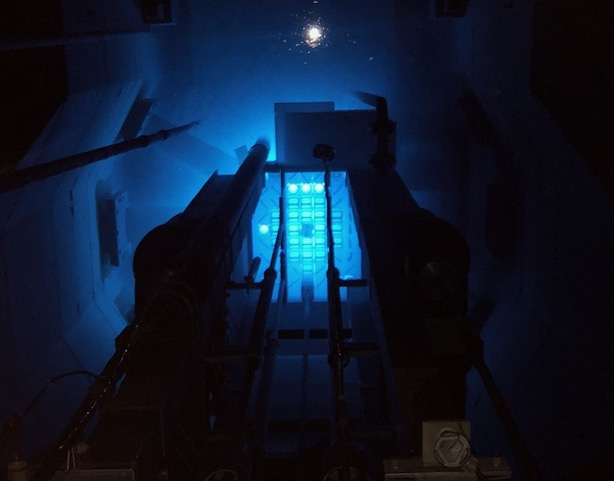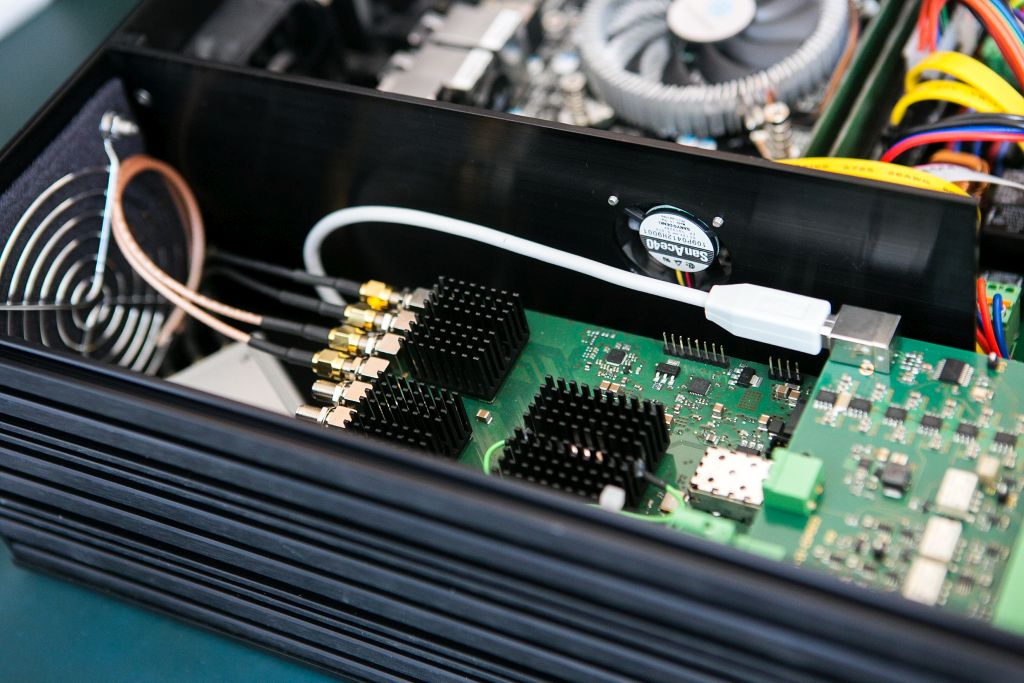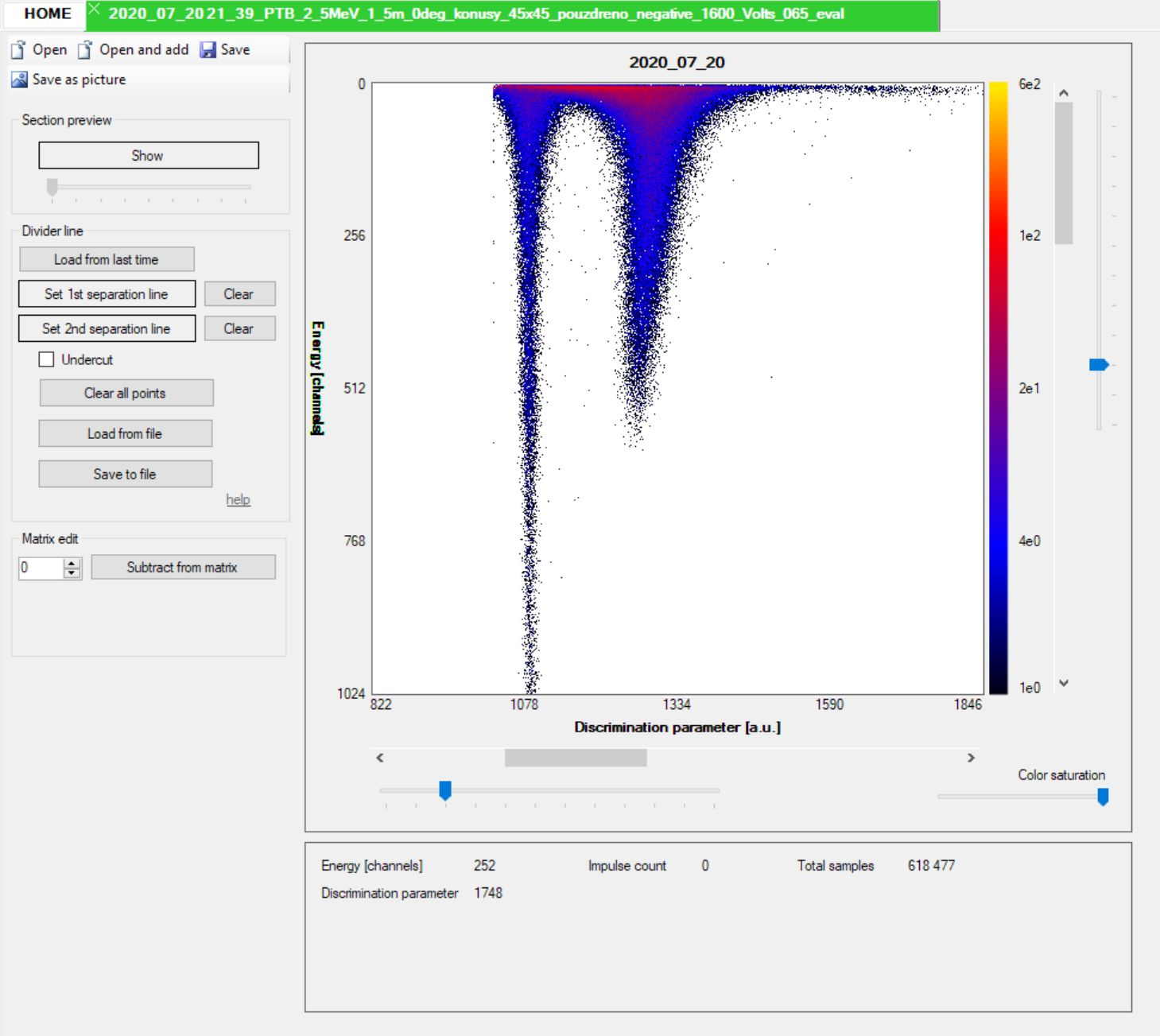# Spectrometer

We work on algorithms and digitization parts for spectrometric systems for measuring neutron fluxes.

The two-parameter spectrometric system NGA-01 is fully digitized and is now able to process up to 500 000 impulse responses per second. In the described case, the tapered active voltage divider was used for the photomultiplier tube. This type of divider has better output linearity than previously used passive resistance, where the problem with non-linearity could exist even from relatively low rates about from 10^4 impulses per second. Solving the non-linearity problem with active voltage divider based on MOSFETs also solves the problem of non-linearity depending on the amplitude of the signal.

A large dynamic range of input signal is required for measurement. Therefore, the input analog signal from the photomultiplier is divided into two branches with different ratio of amplification (1:8). The signal is then digitized by separate analog to digital converters with 12-bit resolution. A high-pass filter is used for better resolution. The difference in amplification increases the signal-to-noise ratio. Analog-to-digital converters operating at a sampling frequency of 500 MHz are used, and digital signal processing is implemented in the field-programmable gate array (FPGA). Therefore, it can process all the data flow from both analog to digital converters with negligible dead time in the digital part. Problems at high frequencies (> 10^5 pulses per second) are superpositions on signals that can affect the resulting values. These pulses are largely filtered.

Pulse Shape Discrimination (PSD) unit is used to distinguish the type of the detected particle by analyzing the pulse shape (discrimination integral), whereas particle energy is evaluated from the integral of the whole response (energy integral). The PSD value is computed inside the FPGA by an integration method that uses the comparison of the area delimited by part of a trailing edge of the measured response with the area delimited by the whole response.